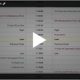# How Can You Measure Risk in Treasuries?

Interest Rate Education Homepage

When it comes to measuring risk for fixed income (rates) traders and portfolio managers, they tend to use one or two yardsticks, value of a basis point and modified duration.

Value of a basis point (VBP), also known as basis point value (BPV), or, for U.S. dollar products, dollar-value of an 01 (DV01),is the financial effect of a 0.01% (one-basis point) change in that instrument’s yield.

For example, if a 10-Year note is current 1.30% yield to maturity with a DV01 of \$859 per million par value and the yield goes up by 0.01 to 1.31%, we would expect the financial value of that note to drop by \$859 per million par.

DV01

One can identify the DV01 of individual securities or an average DV01 of a whole portfolio. DV01s tend to get larger as you move out the yield curve.

For example, a 2-Year U.S. Treasury note may have a DV01 of \$185 per million par while a 30-year Treasury bond may have a DV01 or \$2,131 per million par.

Modified Duration

Modified duration represents the financial effect as a percentage gain or loss to a 1.0% (100 basis points) change in underlying yield.

For example, consider our previously mentioned 10-Year note: if its duration was 8.95 years and yields move higher from 1.30% to 2.30%, or by 1.0%, we would expect the value to fall by 8.95% in value.

Treasury DV01
Ultra 30-Year \$289.34
30-Year \$213.14
Ultra 10-Year \$115.84
10-Year \$76.55
5-Year \$47.94
2-Year \$36.97

In general, the longer the maturity, the greater the price sensitivity and risk. Duration measures this risk precisely.

Traders and portfolio managers routinely refer to your position or portfolio in basis point value and modified duration terms.

Implied Basis Point Value and Implied Duration

U.S. Treasury futures can also be referred to in implied duration and implied basis point value terms.

To look more closely at the BPV and modified duration of a futures contract, we must first go back to the concept of a U.S. Treasury futures contract’s cheapest-to-deliver (CTD) security. You may recall from previous modules a U.S. Treasury futures contract’s CTD security is the eligible bond or note that is most financially efficient for the short position to deliver to the long position at contract expiration. Very few market participants go all the way to delivery, in fact the number is quite low (usually less than 5% of open interest).

The reasons we want to know about the CTD security is two-fold: contracts trade like their CTD security and  we will use the CTD security and its conversion factor to arrive at that contracts implied BPV.

Once we know a U.S. Treasury’s CTD security we can determine that security’s BPV per \$100,000 face value (or \$200,000 face value in the case of 2-Year note futures). We use \$100,000 because, with the exception of 2-year notes which have an underlying face value of \$200,000 per contract, U.S. Treasury contracts have an underlying face value of \$100,000 per contract.

Once we know a contract’s CTD we can determine its BPV; and using that security’s conversion factor (CF) and some simple mathematics, arrive at the implied BPV.

Assume we have a 5-Year Note futures contract and its CTD security is the 1.375% of May 31, 2021 with a BPV per \$100,000 of \$42.45 and a conversion factor of 0.8317.

To arrive at the BPV, we take the BPV of CTD and divide it by its conversion factor:

BPVcontract = BPV ctd ÷ CF

For our example, BPVcontract = 42.45 / 0.8317 = \$51.04 per contract.

Once we have the implied BPVs for the U.S. Treasury futures contracts we can use them to calculate appropriate dollar-weighted hedge ratios versus a cash security of portfolio. We could also calculate the spread ratios between futures contracts so we can construct dollar-weighted yield curve trades.

Interest rate traders and managers of risk use basis point value and modified duration to measure their market risk. Futures contracts based on U.S. Treasury securities can also be referred to in implied basis point value and implied modified duration with a little knowledge of how the contracts price and behave and some simple math. Knowing the contract’s CTD is the starting point.

If you have questionsor schedule an online review .

Regards,

—————————————————————-

Privacy Notice

Disclosure